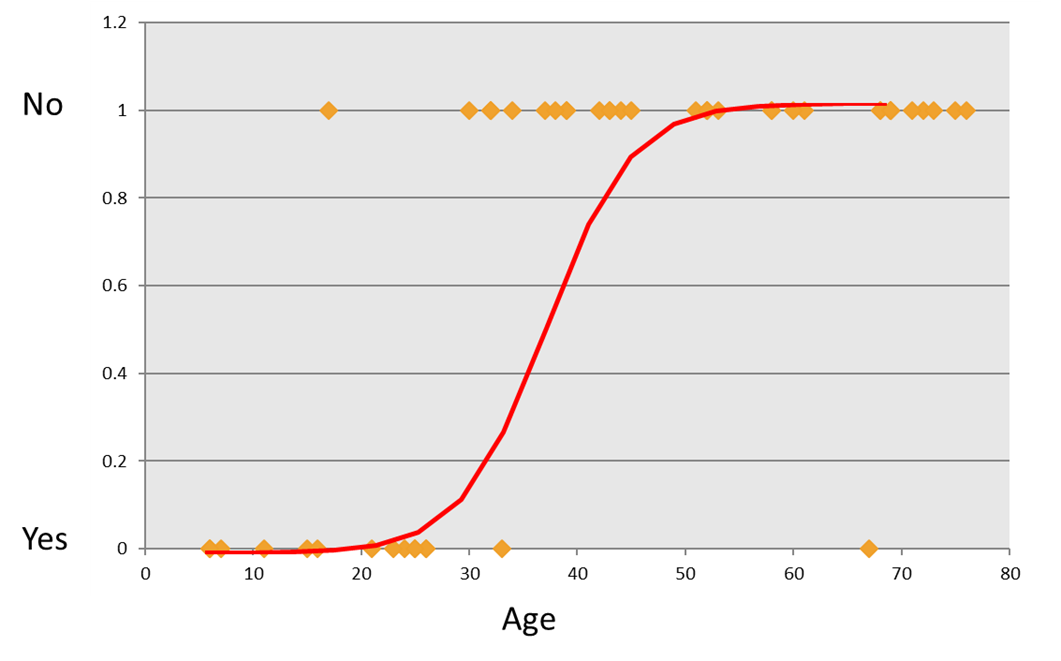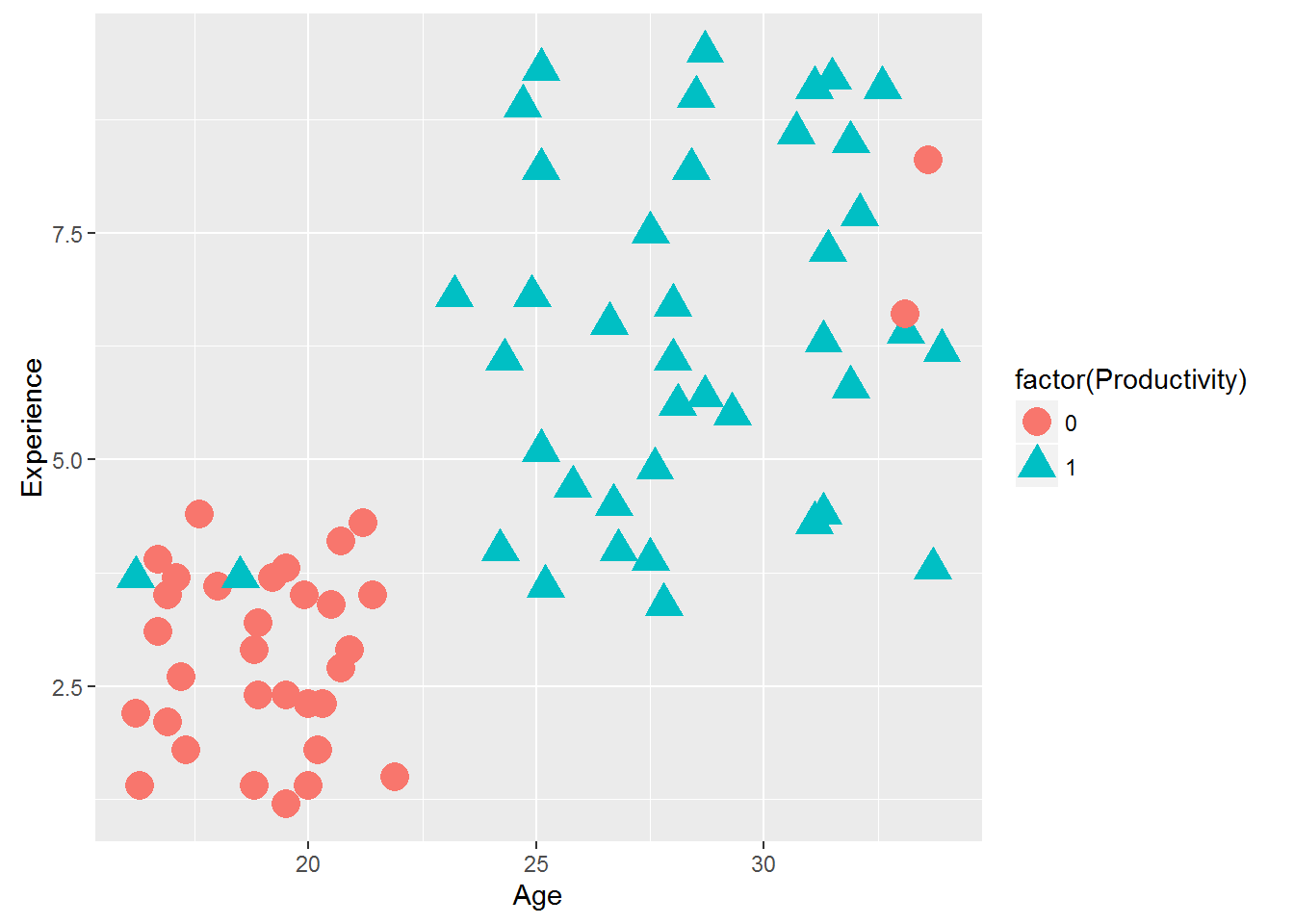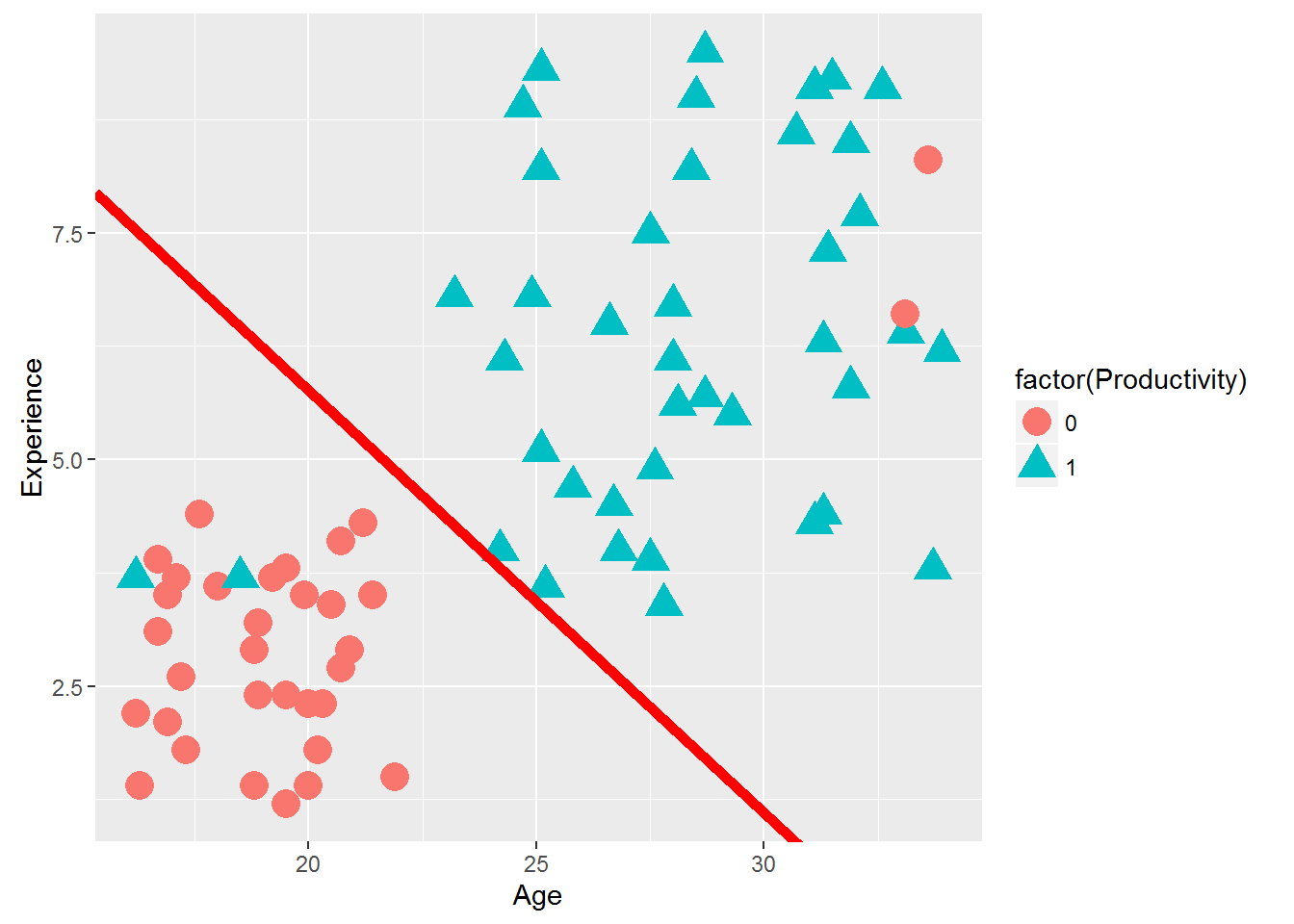• No products in the cart.

# 203.5.1 Neural Networks : A Recap of Logistic Regression

##### Before we dig into Neural Networks.

In previous section, we studied about Cross Validation

In this post we will just revise our understanding of how logistic regression works, which can be considered a building block for a neural network.

Contents

• Neural network Intuition
• Neural network and vocabulary
• Neural network algorithm
• Math behind neural network algorithm
• Building the neural networks
• Validating the neural network model
• Neural network applications
• Image recognition using neural networks

### Recap of Logistic Regression

• Categorical output YES/NO type
• Using the predictor variables to predict the categorical output### LAB: Logistic Regression

• Dataset: Emp_Productivity/Emp_Productivity.csv
• Filter the data and take a subset from above dataset . Filter condition is Sample_Set<3
• Draw a scatter plot that shows Age on X axis and Experience on Y-axis. Try to distinguish the two classes with colors or shapes (visualizing the classes)
• Build a logistic regression model to predict Productivity using age and experience
• Finally draw the decision boundary for this logistic regression model
• Create the confusion matrix
• Calculate the accuracy and error rates

### Solution

``Emp_Productivity_raw <- read.csv("C:\\Amrita\\Datavedi\\Emp_Productivity\\Emp_Productivity.csv")``
• Filter the data and take a subset from above dataset . Filter condition is Sample_Set<3
``````Emp_Productivity1<-Emp_Productivity_raw[Emp_Productivity_raw\$Sample_Set<3,]

dim(Emp_Productivity1)``````
``##  74  4``
``names(Emp_Productivity1)``
``##  "Age"          "Experience"   "Productivity" "Sample_Set"``
``head(Emp_Productivity1)``
``````##    Age Experience Productivity Sample_Set
## 1 20.0        2.3            0          1
## 2 16.2        2.2            0          1
## 3 20.2        1.8            0          1
## 4 18.8        1.4            0          1
## 5 18.9        3.2            0          1
## 6 16.7        3.9            0          1``````
``table(Emp_Productivity1\$Productivity)``
``````##
##  0  1
## 33 41``````
• Draw a scatter plot that shows Age on X axis and Experience on Y-axis. Try to distinguish the two classes with colors or shapes (visualizing the classes)
``````library(ggplot2)
ggplot(Emp_Productivity1)+geom_point(aes(x=Age,y=Experience,color=factor(Productivity),shape=factor(Productivity)),size=5)``````– Build a logistic regression model to predict Productivity using age and experience

``````Emp_Productivity_logit<-glm(Productivity~Age+Experience,data=Emp_Productivity1, family=binomial())
Emp_Productivity_logit``````
``````##
## Call:  glm(formula = Productivity ~ Age + Experience, family = binomial(),
##     data = Emp_Productivity1)
##
## Coefficients:
## (Intercept)          Age   Experience
##     -8.9361       0.2763       0.5923
##
## Degrees of Freedom: 73 Total (i.e. Null);  71 Residual
## Null Deviance:       101.7
## Residual Deviance: 46.77     AIC: 52.77``````
``coef(Emp_Productivity_logit)``
``````## (Intercept)         Age  Experience
##  -8.9361114   0.2762749   0.5923444``````
``````slope1 <- coef(Emp_Productivity_logit)/(-coef(Emp_Productivity_logit))
intercept1 <- coef(Emp_Productivity_logit)/(-coef(Emp_Productivity_logit)) ``````
• Finally draw the decision boundary for this logistic regression model
``````library(ggplot2)
base<-ggplot(Emp_Productivity1)+geom_point(aes(x=Age,y=Experience,color=factor(Productivity),shape=factor(Productivity)),size=5)
base+geom_abline(intercept = intercept1 , slope = slope1, color = "red", size = 2) #Base is the scatter plot. Then we are adding the decision boundary``````– Create the confusion matrix

``````predicted_values<-round(predict(Emp_Productivity_logit,type="response"),0)
conf_matrix<-table(predicted_values,Emp_Productivity_logit\$y)
conf_matrix``````
``````##
## predicted_values  0  1
##                0 31  2
##                1  2 39``````
• Calculate the accuracy and error rates
``````accuracy<-(conf_matrix[1,1]+conf_matrix[2,2])/(sum(conf_matrix))
accuracy``````

```##  0.9459459 ```

21st June 2017
0 responses on "203.5.1 Neural Networks : A Recap of Logistic Regression"